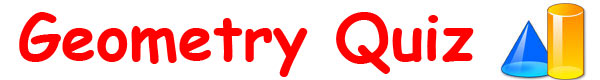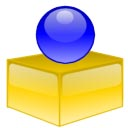Geometry Quiz for KidsHow much do you know about geometry? Try this fun geometry quiz for kids and find out!Put your knowledge to the test with a range of questions & answers related to dimensions, angles, lengths and shapes such as triangles, circles and squares. Answer as many of the ten questions as you can, scroll down to the bottom of the page and see how many you got correct. Sponsored Links

 1. How many sides does a hexagon have? 2. True or false? A parallelogram features three pairs of parallel sides. 3. The internal angles of an equilateral triangle all measure how many degrees? 4. How many points are there on a pentagram? 5. True or false? A concave shape bends inwards. 6. The distance from the center of a circle to its edge is called what? 7. How many sides of equal length does a square have? 8. True or false? A scalene triangle has two sides of equal length. 9. How many dimensions does a semicircle feature? 10.A trapezoid (trapezium) features how many pairs of parallel sides?